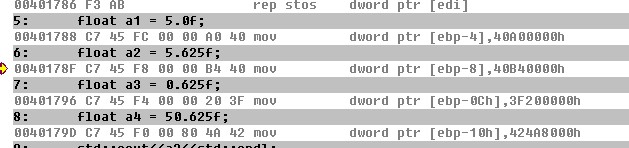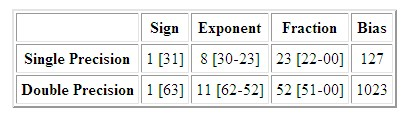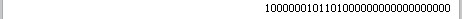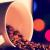# 解惑IEEE754 浮点数内存布局

这几天，群里看到有初学者在询问float的相关内容。大家给他的答复就是自己网上搜内存布局的帖子， 我事后也看了下，发现很多帖子写的都很迷糊。今天，我想和各位同学一起深入的看下754浮点布局状况。下面是我们今天需要调试的程序，由于程序很短所以直接贴图。### The Sign Bit

The sign bit is as simple as it gets. 0 denotes a positive number; 1 denotes a negative number. Flipping the value of this bit flips the sign of the number.

### The Exponent

The exponent field needs to represent both positive and negative exponents. To do this, abias is added to the actual exponent in order to get the stored exponent. For IEEE single-precision floats, this value is 127. Thus, an exponent of zero means that 127 is stored in the exponent field. A stored value of 200 indicates an exponent of (200-127), or 73. For reasons discussed later, exponents of -127 (all 0s) and +128 (all 1s) are reserved for special numbers.

For double precision, the exponent field is 11 bits, and has a bias of 1023.

### The Mantissa

The mantissa, also known as the significand, represents the precision bits of the number. It is composed of an implicit leading bit and the fraction bits.

To find out the value of the implicit leading bit, consider that any number can be expressed in scientific notation in many different ways. For example, the number five can be represented as any of these:

```        5.00 × 100
0.05 × 102
5000 × 10-3
```

In order to maximize the quantity of representable numbers, floating-point numbers are typically stored innormalized form. This basically puts the radix point after the first non-zero digit. In normalized form, five is represented as 5.0 × 100.

A nice little optimization is available to us in base two, since the only possible non-zero digit is 1. Thus, we can just assume a leading digit of 1, and don't need to represent it explicitly. As a result, the mantissa has effectively 24 bits of resolution, by way of 23 fraction bits.

### Putting it All Together

So, to sum up:

1. The sign bit is 0 for positive, 1 for negative.
2. The exponent's base is two.
3. The exponent field contains 127 plus the true exponent for single-precision, or 1023 plus the true exponent for double precision.
4. The first bit of the mantissa is typically assumed to be 1.f, where f is the field of fraction bits.

1. 就是 符号位的那点破事

2 就是指数的底数为2

3 这个它说的很模糊 什么叫指数加上一个真指数 太操蛋，（他给的例子中200超出了范围所以-127，而这个范围是多少我想最后的结果肯定不能超过8个1）

4 这也是非常关键的一点 ， 第一位是从1开始的，因为是二进制数的原因我在下面进行演示。

5.0 的 二进制 我们先把整数部分 化为 二进制 为 1015.625f

0.625 转为 二进制 。。。。什么？不会？ 好吧，我来补一课。 小数部分10进制转2进制 是*2 取整，然后减去整数部分继续乘2

0.25*2 = 0.5 取 0 减0

0.5*2 = 1.0 取1 OVER

40B400000